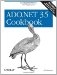# Exporting the Results of a Query to an Array

Problem

You need to export the results of a query to an array in a manner similar to the GetRows( ) method of the ADO Recordset .

Solution

Write a routine to mimic the functionality of the ADO Recordset 's GetRows( ) method.

The sample code contains an event handler and a method:

Go Button.Click

Sets up the sample by creating a DataTable containing the Orders table from Northwind. The GetRows( ) method in this solution is called to convert the DataTable into an array similar to one that is generated by the GetRows( ) method of the ADO Recordset . The contents of the array are displayed to a text box on the form.

GetRows( )

This method mimics the functionality of the GetRows( ) method of the ADO Recordset . First, an object array is created to hold the rows and columns in the table. The number of columns in the array is set to hold either all of the columns in the table or the subset of columns defined by the optional string array of column names . The number of rows in the table is set to hold either all of the rows in the table or the subset defined by the optional start row and row count arguments. The method then iterates over the collection of rows and columns in the table and sets the values in the array to the field values.

The C# code is shown in Example 5-14.

```// Namespaces, variables, and constants
using System;
using System.Configuration;
using System.Text;
using System.Data;
using System.Data.SqlClient;

// Table name constants
private const String ORDERS_TABLE = "Orders";

// . . .

private void goButton_Click(object sender, System.EventArgs e)
{
// Fill the Order table.
ConfigurationSettings.AppSettings["Sql_ConnectString"]);
DataTable dt = new DataTable(ORDERS_TABLE);
da.Fill(dt);

StringBuilder sb = new StringBuilder( );

Array a = GetRows(dt, -1, -1, null);

// Iterate over the rows of the array.
for(int iRow = 0; iRow < a.GetLength(0); iRow++)
{
// Iterate over the columns of the array.
for(int iCol = 0; iCol < a.GetLength(1); iCol++)
{
sb.Append(a.GetValue(iRow, iCol).ToString( ) + "	");
}
sb.Remove(sb.Length - 2, 2);
sb.Append(Environment.NewLine);
}

resultTextBox.Text = sb.ToString( );
}

private Array GetRows(DataTable dt, int rowCount, int startRow,
String[] colName)
{
// If column names are specified, ensure that they exist in the table.
if (colName != null)
{
for (int i = 0; i < colName.Length; i++)
{
if (!dt.Columns.Contains(colName[i]))
throw(new ArgumentException("The column " +
colName[i] +
" does not exist in the table."));
}
}

// If no columns were specified, set the number of columns to the
// number of columns in the table; otherwise, set the number of
// columns to the number of items in the specified columns array.
int nCols = (colName == null) ? dt.Columns.Count : colName.Length;

// The table row to start exporting
// Set to 1 if less than 1 is specified.
startRow = (startRow < 1) ? 1 : startRow;

// The number of rows to export calculated as the number of rows in
// the table less the starting row number
// If the starting row is specified as greater than the number of
// rows in the table, set the number of rows to 0.
int nRows = Math.Max((dt.Rows.Count - startRow) + 1, 0);
// If the number of rows to export is specified as greater than 0,
// set the number of rows to export as the lesser of the number
// specified and the number of rows calculated in the table
// starting with the specified row.
if (rowCount >= 0)
nRows = Math.Min(nRows, rowCount);

// Create an object array to hold the data in the table.
Array a = Array.CreateInstance(typeof(object), nRows, nCols);

// Iterate over the collection of rows in the table.
for(int iRow = startRow - 1; iRow < startRow - 1 + nRows; iRow++)
{
if (colName == null)
{
// Iterate over the collection of columns in the table.
for(int iCol = 0; iCol < dt.Columns.Count; iCol++)
{
// Set the cell in the array.
a.SetValue(dt.Rows[iRow][iCol], iRow, iCol);
}
}
else
{
for(int i = 0; i < colName.Length; i++)
{
// Set the cell in the array.
a.SetValue(dt.Rows[iRow][colName[i]],
iRow - startRow + 1, i);
}
}
}

return a;
}
```

Discussion

There is no ADO.NET DataTable method that works like the GetRows( ) method of the ADO Recordset or method that converts the Recordset into a two-dimensional array.

This solution presents an ADO.NET method, which is also called GetRows( ) , that duplicates the functionality of the ADO GetRows( ) method. The prototype for the ADO.NET method is:

```Object[][] tableArray = GetRows(DataTable dt, Integer rowCount, Integer startRow,
String[] colName);
```

Parameters

tableArray

Returns an array of field values corresponding to the values in the columns and rows selected from the table.

dt

The DataTable to convert to the array.

rowCount

The number of rows to export to the array.

startRow

The row number of the first row to export.

fields

A string array containing the names of the columns to export. If this parameter is null, all columns are exported.

Unlike the ADO method, columns cannot be specified by their ordinal values. An overloaded GetRows( ) method that accepts the column ordinals rather than names could be written to do this.

## Maintaining Database Integrity